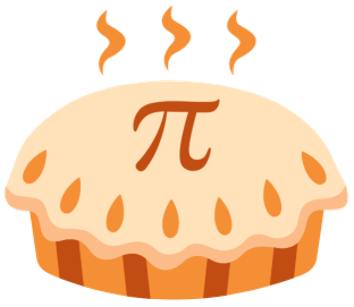# Can I Get Some Pi?

March 16, 2023

Pi is a mathematical constant. It can be defined as the ration of a circle’s circumference to its diameter. A circle’s diameter is the measurement from one side to another. The circumference is the measurement around the outside of the circle. If we draw a circle with a diameter of 1, then the circumference is 3.14159265...a number known as Pi. Pi is written using the Greek symbol π.

Materials:

- 100 Toothpicks

- Large Poster Board

- Paper

- Pencil

- Ruler

Procedure:

1. Using your ruler, measure the length of a toothpick and double it. This number is important! Write it down for the next step.

2. Using the number you just calculated and your ruler, start at one end of your poster board and make a vertical line each time you measure your number.

3. Next, randomly dump your toothpicks onto the board. You want to make sure they are spread out and not clumped together. One way to make this easier is to put them all in a cup and pour them out onto the board.

4. Count how many toothpicks are touching or crossing the lines on your board.

5. Math time! You are going to use this formula to calculate a number that is close to pi.

6. To calculate the average from your trials, add all of your results together and then divide by the number of trials you did. Use the table below to help you with this calculation.

What’s happening? A circle’s diameter is the measurement from one side to  another, the circumference is the measurement around the outside of the circle, and the radius is the measurement from the center to one side. This experiment is one of the oldest problems in the field of geometric probability.

The experiment demonstrates the relationship between a circle’s radius, diameter,  circumference, and the value of pi. The Law of Large Numbers states the more trials you do, the closer your average will be to the overall average – or in our case, π.